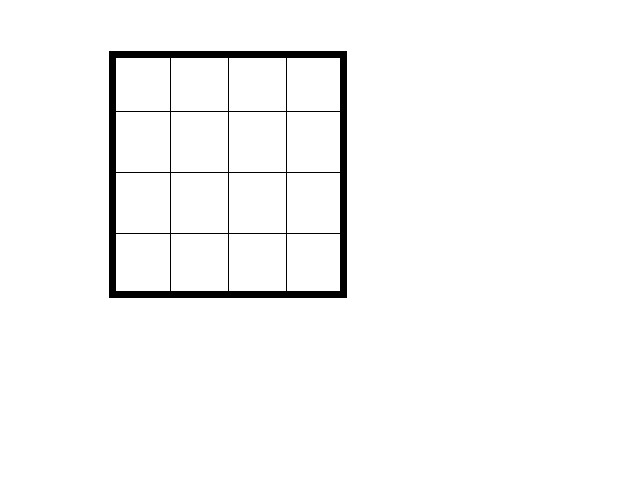# Lecture 3: Make Your Own KenKen

In this section you will get a chance to make your own KenKen puzzle. There is no set "good" way to do this, you just have to make sure that in the end your puzzle has exactly one answer and can be solved with no guessing using only the rules of KenKen. Here are some suggestions for figuring out how to make a puzzle that has exactly one of each of the numbers 1,2,3,4 in each row and column and can be solved using the rules of KenKen without guessing.

Start with an empty grid and put in the numbers 1,2,3,4 in the cells so that each row and column has exactly one of each number.Now start adding bold shapes and picking operations for the bold shapes. Once you have picked an operation you know what the number accompanying the shape and operation must be, because you know what the entries are and you know the operation. This is where things get tricky, since you need to ensure that there is exactly one solution to the puzzle that can be figured out using only the rules of KenKen. Now, you erase your numbers and you have a puzzle.

A note of caution about subtraction and division. The order that those operations are done in matters, and while we have the rule that your operation only needs to work out in some order, it means that you cannot have three or more squares in some bolded area without significant ambiguity. For example, we have the problem that 4/(2/2)=4, but (4/2)/2=1, and this introduces a lot of confusion into what should be done in a puzzle. In most cases the best thing to do is to just avoid bolded areas with subtraction or division and more than two cells.

How do you check that your puzzle has only one solution? One choice is to give it to a friend. If she or he can do it without guessing then you are safe. If, however, they can find more than one solution, you need to do some rearranging.

This work was made possible through a grant from the National Science Foundation.